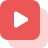Home » About SSC » SSC Study Materials » Divisibility Test Of 7 Or 13

# Divisibility Test Of 7 Or 13

This article is going to focus on the details regarding the test of divisibility of 7 or 13, with examples .The article will further mention important details about the divisibility test of 7 and 13.

# Divisibility Test Of 7 Or 13

### What Is Divisibility in layman terms?

Divisibility in maths relates to a number’s ability to be equally divided by another integer without leaving a remainder. For instance, the divisibility of 100 by 10 is obvious. A massive chunk of cake’s divisibility implies that it could be cut into smaller bits and sold individually, while a pizza’s divisibility is obvious once you chop it to equal sizes and serve equal portions to each of your guests.

The divisibility rules like the divisibility test of 7 or 13, are sort of techniques that help in determining if a given number is divisible by another number. The usual division process takes a lot of work if the given number is large. Divisibility tests help make that large number easier to handle.

These sets of rules that help the users or readers figure out if a number can be divisible by another, by merely looking at its digits, are named as divisibility rules or tests.

Divisibility of 7 Or 13:

The  divisibility test of 7  says that when an integer is divided by 7, the final digit must be multiplied with 2 and then removed from the remainder of the value, preserving the very last digit. It should be divisible by 7  if the difference is 0 or a multiple of 7. The Divisibility rule or law, also known as the Divisibility test, allows us to determine if a value is totally divisible by some other value without having to use the division process.

The divisibility test of 13 says that if an integer’s one’s place digit gets multiplied with 4 as well as the result is added to the remainder of the value, the result seems to be either 0 or a multiple of 13. In short , the sum generated by multiplying the unit digits by 4 then adding the result to the remaining number after removing the one’s place digit results in a new number. If the new number is divisible by 13 then the whole number is divisible by 13.

Divisibility Rule For Bigger Numbers:

For smaller integers, the divisibility test of 7 is simple to test. For larger numbers, meanwhile, we use the divisibility test of 7. When dealing with greater numbers, simply continue the procedure of using the divisibility test unless we are confident that now the value must be divisible by seven.

For the divisibility test of 13, multiply the unit position digits by 4, now add the result of the integer to the number left after striking out the unit digit. If the result seems to be a Zero or multiples of 13, the integer is divisible with thirteen.

Examples Regarding Divisibility By 7 Or 13:

Example One:

Verify whether the number 56 is divisible by 7.

Solution:

Here with the number 56, when the digit in one’s position is multiplied by two it gives the output,

2 x 6 = 12

The difference between the new number and the rest of the numbers after striking out the one’s place digit is

12-5 = 7

The number 7 is divisible by seven.

As a result, the value 56 is divisible by 7.

Example Two:

Verify whether the number 804 is divisible by 7.

Solution:

In the given value 804, the digit in one’s position is multiplied by two.

2 x 4 = 8

The difference between the new number that is ‘8’ and the rest of the numbers after striking out the one’s place digit is

80 – 8 = 72

The number 72 isn’t divisible by seven.

As a result, the value 804 isn’t divisible by 7.

Examples for divisibility of 13

Example one:

Verify to see if 429 is divisible by 13

Solution:

Following the procedure mentioned above,

Multiply unit place digit with 4

So, 9×4 = 36

Add the result to rest of the numbers

42+36 = 78

The resultant number 78 is divisible by 13, so 429 is divisible by thirteen.

Example two:

Verify to see if 962 is divisible by 13.

Solution:

By following the above mentioned procedure or rule

Multiply unit place digit with 4

So, 2×4 = 8

Add the result to rest of the numbers are as follows

96+8 = 104

The resultant number 104 is divisible by 13, so 962 is divisible by thirteen.

## Conclusion

This article talks about how the divisibility rules work in case of divisibility of 7 and 13. The article shows unique examples and explains them while talking about the divisibility process. This above article elucidates us efficient ways to find answers using a divisibility test of 10.